# Common Core: 7th Grade Math : Multiply and Divide Rational Numbers Using Properties of Operations: CCSS.Math.Content.7.NS.A.2c

## Example Questions

← Previous 1

### Example Question #1 : Multiply And Divide Rational Numbers Using Properties Of Operations: Ccss.Math.Content.7.Ns.A.2c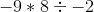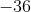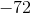Explanation:

Let's work from left to right since it's only multiplication and division. Since there is only one negative number, the product of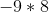is negative which is. Now we have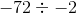. Two negative numbers in a  division problem makes the answer positive.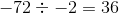### Example Question #1 : Multiply And Divide Rational Numbers Using Properties Of Operations: Ccss.Math.Content.7.Ns.A.2c

Solve: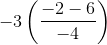Explanation:

When solving this problem remember our orders of operation, or PEMDAS.

PEMDAS stands for parentheses, exponents, multiplication/division, and addition/subtraction. When you have a problem with several different operations, you need to solve the problem in this order and you work from left to right for multiplication/division and addition/subtraction.

Simplify the quantities inside the parentheses.  Then multiply and distribute the coefficient in front.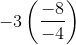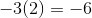### Example Question #1 : Multiply And Divide Rational Numbers Using Properties Of Operations: Ccss.Math.Content.7.Ns.A.2c

Solve: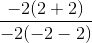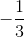Explanation:

When solving this problem remember our orders of operation, or PEMDAS.

PEMDAS stands for parentheses, exponents, multiplication/division, and addition/subtraction. When you have a problem with several different operations, you need to solve the problem in this order and you work from left to right for multiplication/division and addition/subtraction.

Evaluate the quantities inside the parentheses first.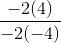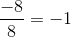### Example Question #491 : Grade 7

Solve: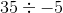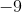Explanation:

We know the following information: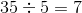However, thechanges our answer, in this particular case. There are a couple of rules that we need to remember when multiplying with negative numbers:

• A negative number divided by a positive number will always equal a negative number, and a positive number divided by a negative number will always equal a negative number.
• A negative number divided by a negative number will always equal a positive number

Thus,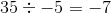### Example Question #301 : The Number System

Solve: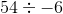Explanation:

We know the following information: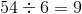However, thechanges our answer, in this particular case. There are a couple of rules that we need to remember when multiplying with negative numbers:

• A negative number divided by a positive number will always equal a negative number, and a positive number divided by a negative number will always equal a negative number.
• A negative number divided by a negative number will always equal a positive number

Thus,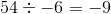### Example Question #1 : Multiply And Divide Rational Numbers Using Properties Of Operations: Ccss.Math.Content.7.Ns.A.2c

Solve: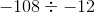Explanation:

We know the following information: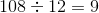In this particular case, do the negative numbers change our answer? . There are a couple of rules that we need to remember when multiplying with negative numbers:

• A negative number divided by a positive number will always equal a negative number, and a positive number divided by a negative number will always equal a negative number.
• A negative number divided by a negative number will always equal a positive number

Thus,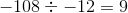### Example Question #8 : Multiply And Divide Rational Numbers Using Properties Of Operations: Ccss.Math.Content.7.Ns.A.2c

Solve: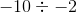Explanation:

We know the following information: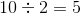In this particular case, do the negative numbers change our answer? . There are a couple of rules that we need to remember when multiplying with negative numbers:

• A negative number divided by a positive number will always equal a negative number, and a positive number divided by a negative number will always equal a negative number.
• A negative number divided by a negative number will always equal a positive number

Thus,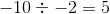### Example Question #1 : Multiply And Divide Rational Numbers Using Properties Of Operations: Ccss.Math.Content.7.Ns.A.2c

Solve: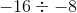Explanation:

We know the following information: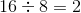In this particular case, do the negative numbers change our answer? . There are a couple of rules that we need to remember when multiplying with negative numbers:

• A negative number divided by a positive number will always equal a negative number, and a positive number divided by a negative number will always equal a negative number.
• A negative number divided by a negative number will always equal a positive number

Thus,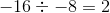### Example Question #10 : Multiply And Divide Rational Numbers Using Properties Of Operations: Ccss.Math.Content.7.Ns.A.2c

Solve: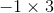Explanation:

We know the following information: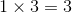However, thechanges our answer, in this particular case. There are a couple of rules that we need to remember when multiplying with negative numbers:

• A negative number multiplied by a positive number will always equal a negative number
• A negative number multiplied by a negative number will always equal a positive number

Thus,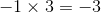### Example Question #491 : Grade 7

Solve: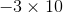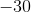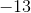Explanation:

We know the following information: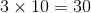However, thechanges our answer, in this particular case. There are a couple of rules that we need to remember when multiplying with negative numbers:

• A negative number multiplied by a positive number will always equal a negative number
• A negative number multiplied by a negative number will always equal a positive number

Thus,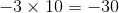← Previous 1

### All Common Core: 7th Grade Math Resources